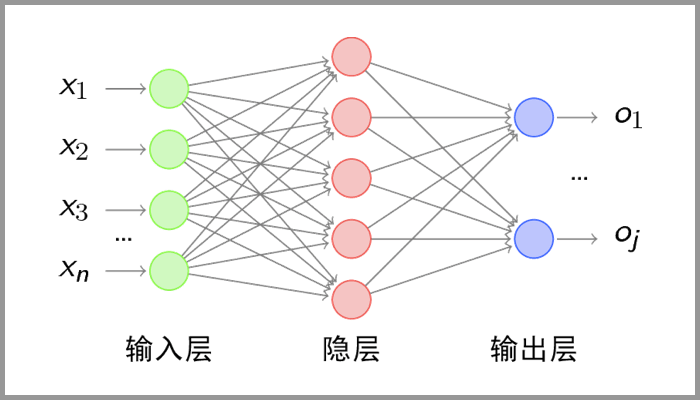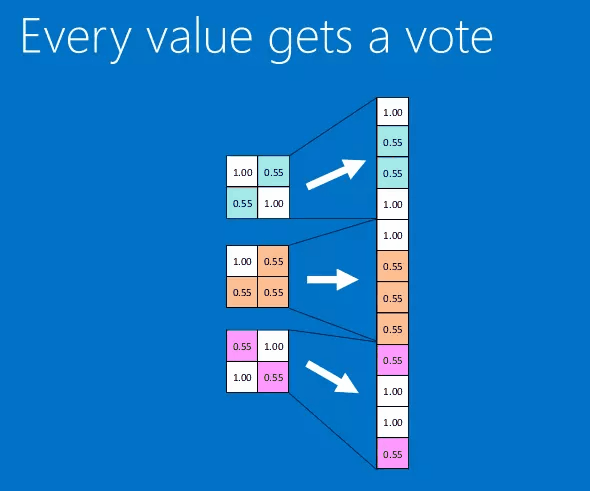## CNN简介

CNN就是受到了人类视觉神经系统的启发，使用卷积核来代替人类中的视野，这样既能够降低计算量，又能够有效的保留图像的特征，同时对图片的处理更加的高效。我们可以想一想前面介绍的DNN网络，它是将一个一个的像素点进行计算的，毋庸置疑，这样必然会带来参数的膨胀，比如说cifar-10中一张32*32*3的图像，第一个隐藏层的中的单个神经元就有$$32 \times 32 \times 3 = 3072$$个权重，似乎挺小的，但是如果图片的像素再大一点，则参数会飞速猛增，同时参数增加的同时就会导致过拟合。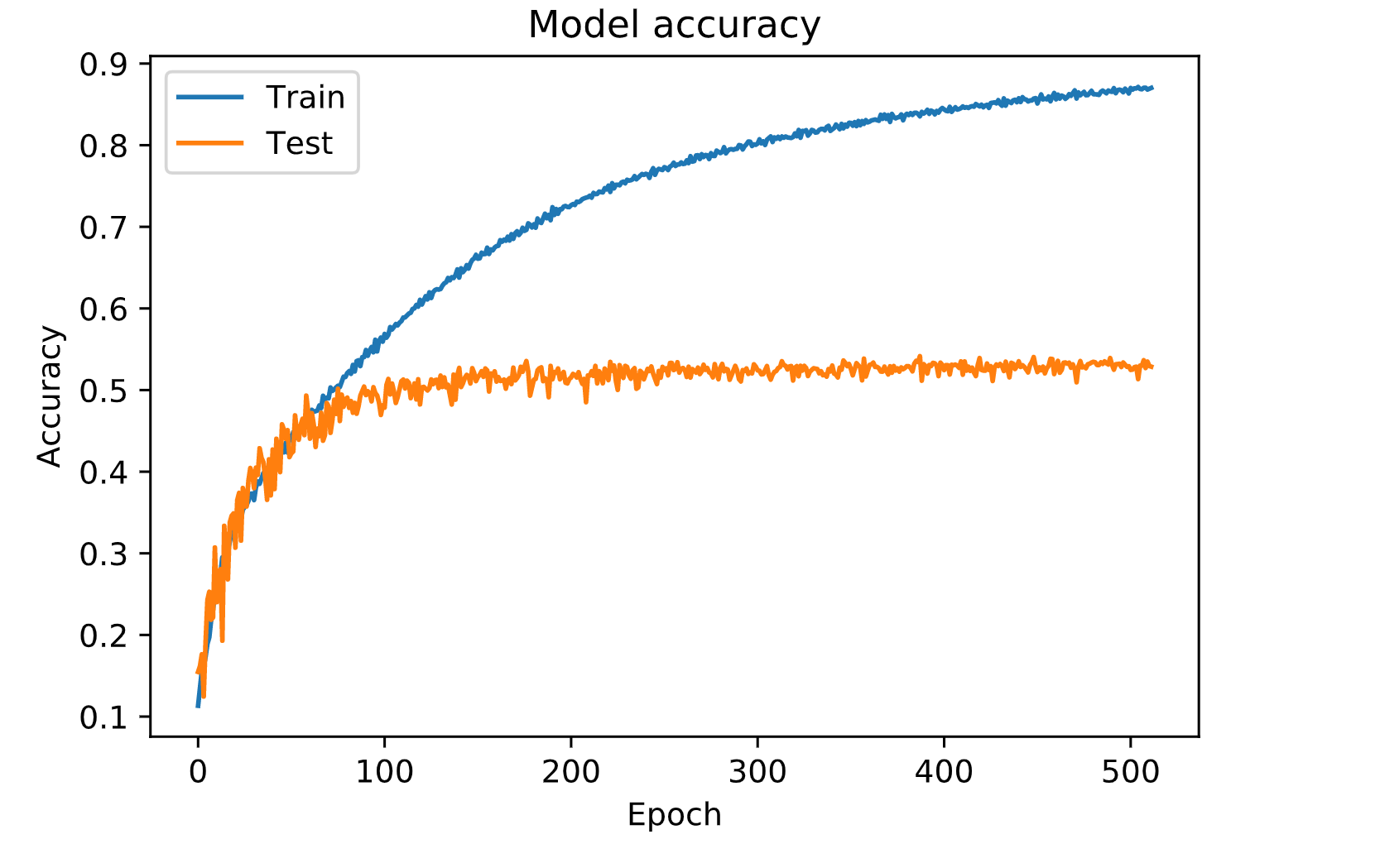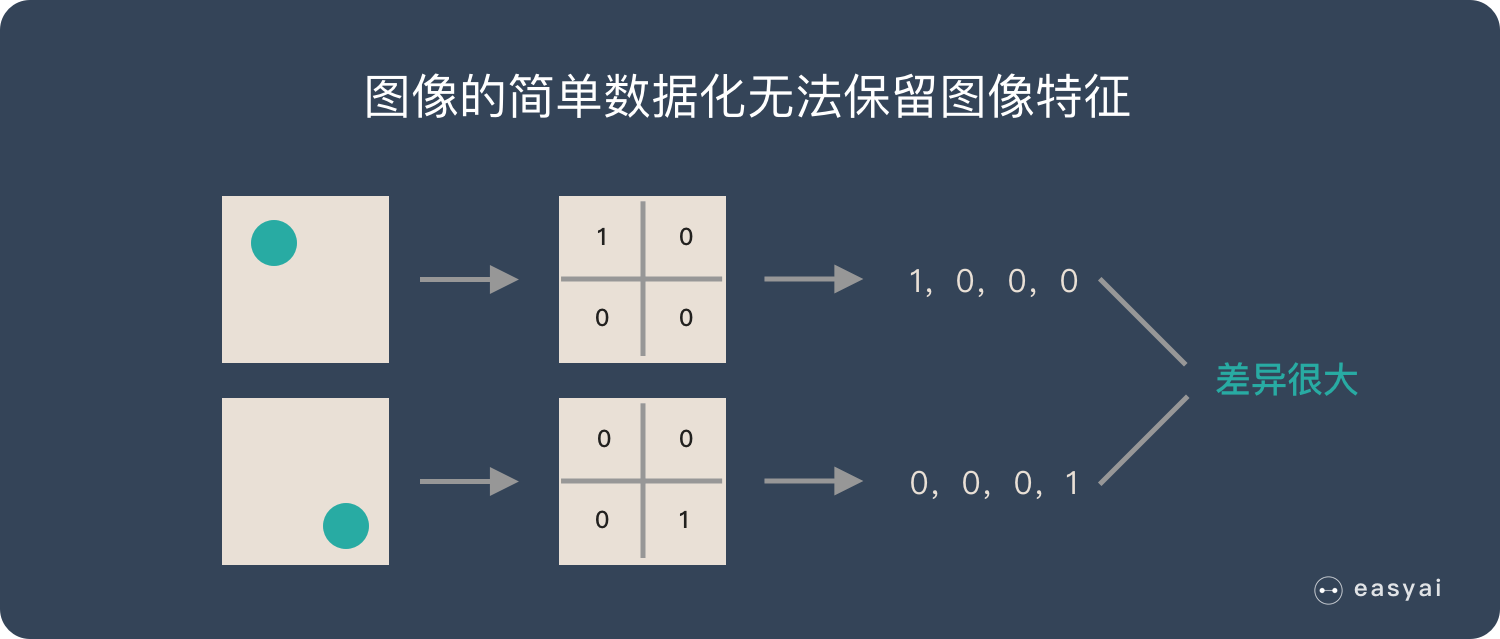• CONV：卷积层，进行卷积计算然后求和。
• Relu：激励层，激励函数为Relu
• POOL：池化层
• FC：全连接层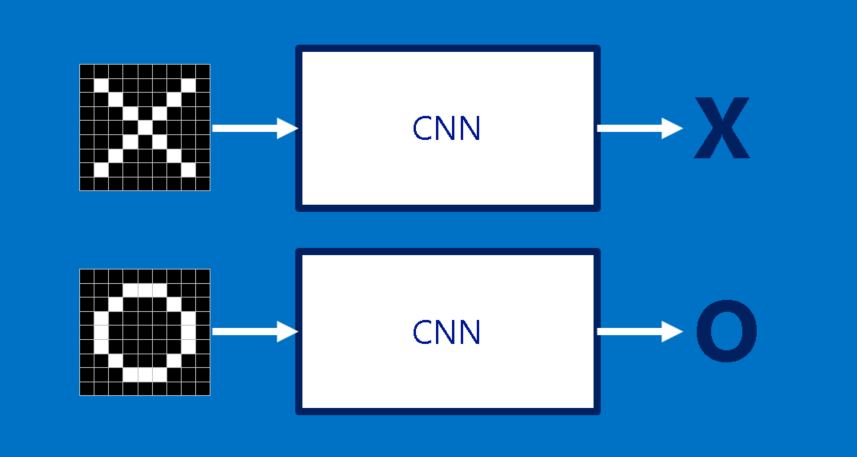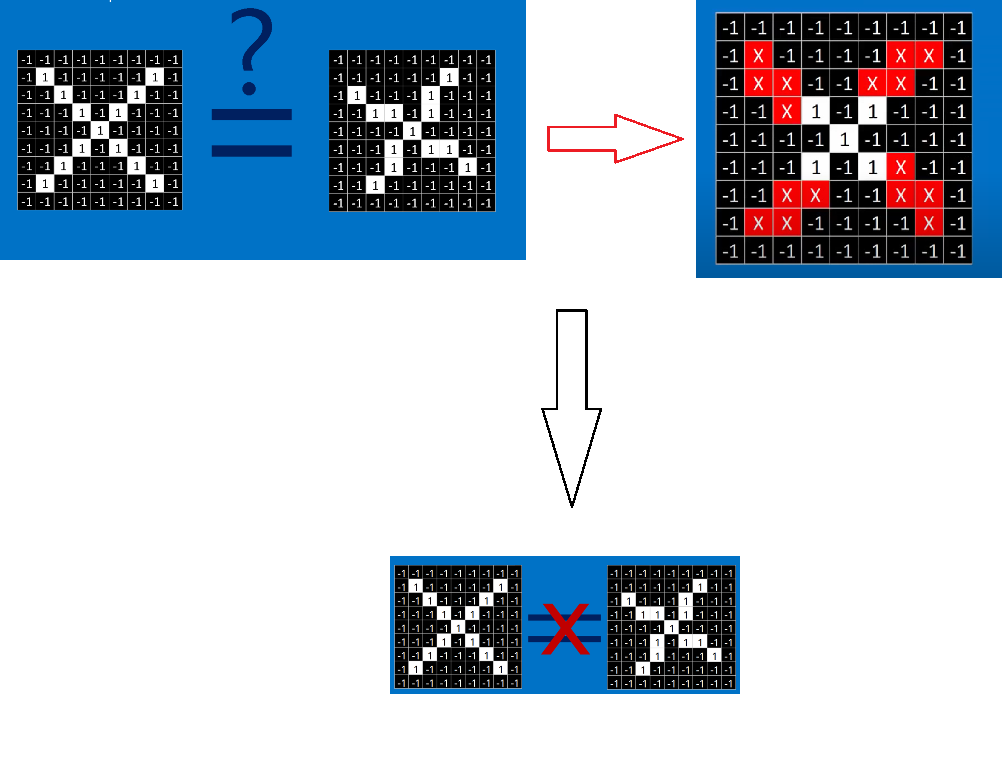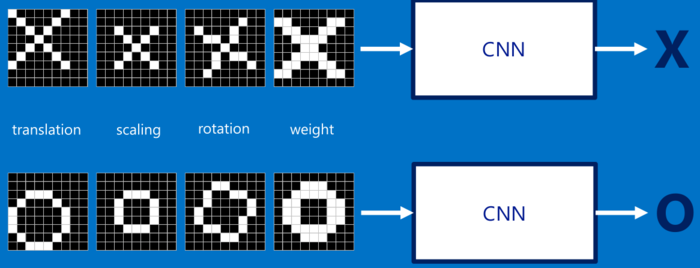so，我们将比较范围由像素点扩大到某一个范围，在CNN模型中，会比较两张图片的的各个局部，这些局部称之为特征（features）。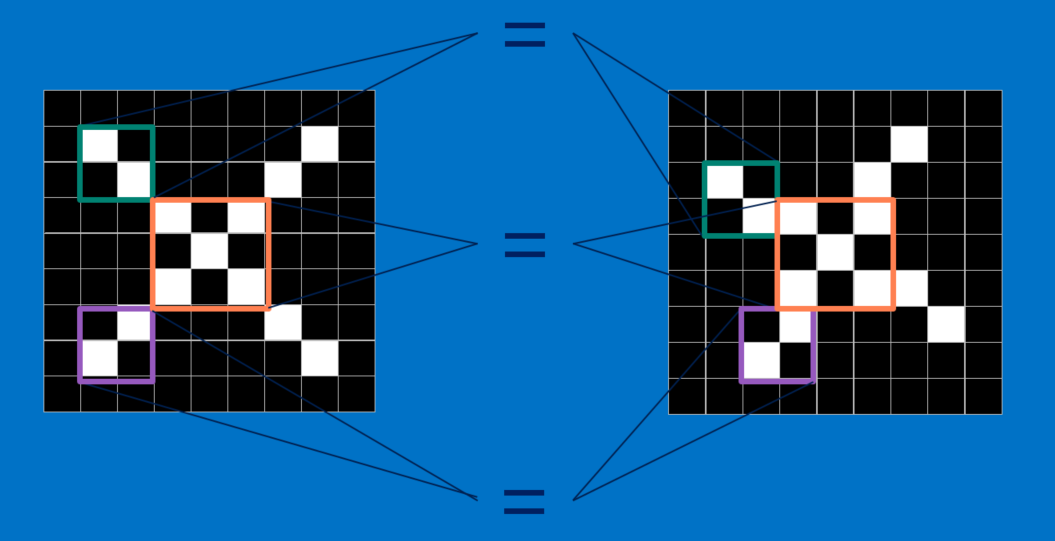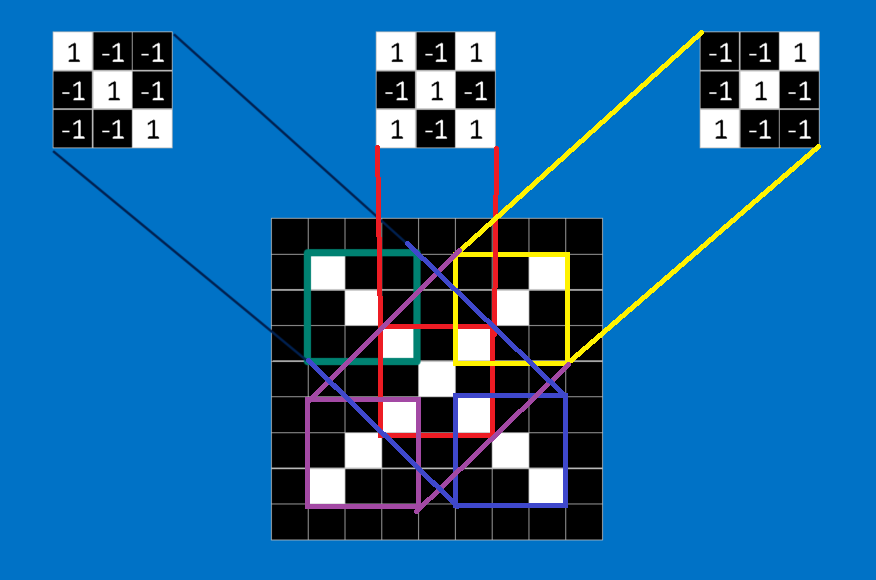## 卷积层(Convolution Layer)

$\frac{(1 \times 1) + (-1\times -1) + (-1\times -1) + (-1\times -1) + (1\times 1) + (-1\times -1)+ (-1\times -1) + (-1\times -1) + (1\times 1)}{9} = 1s$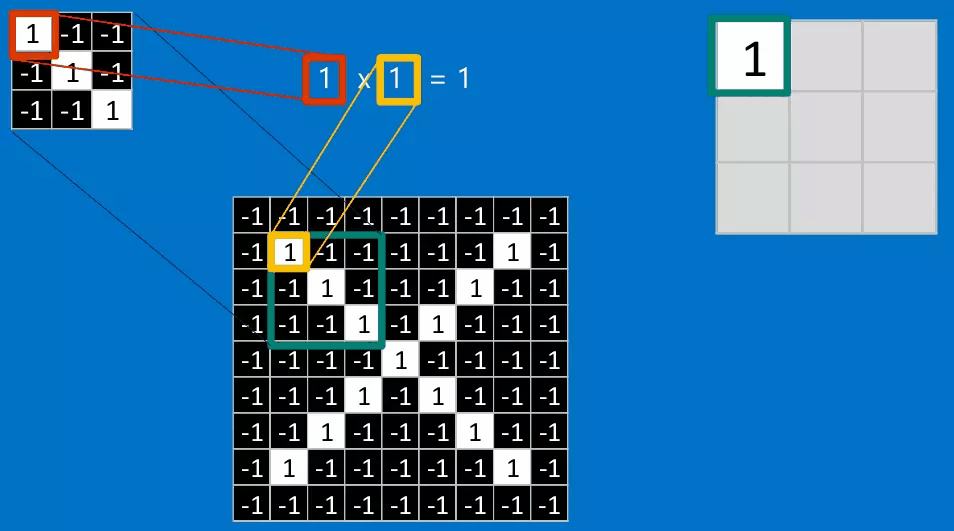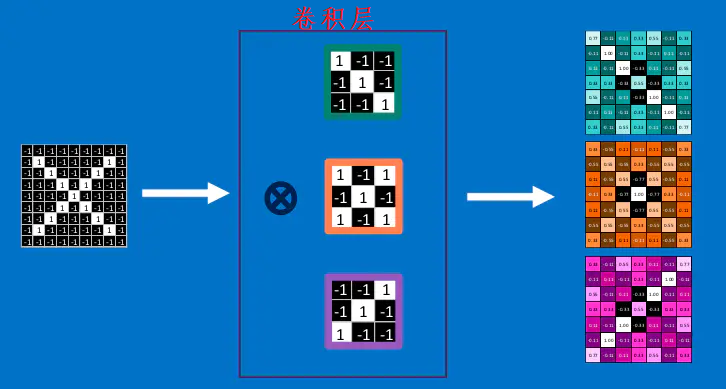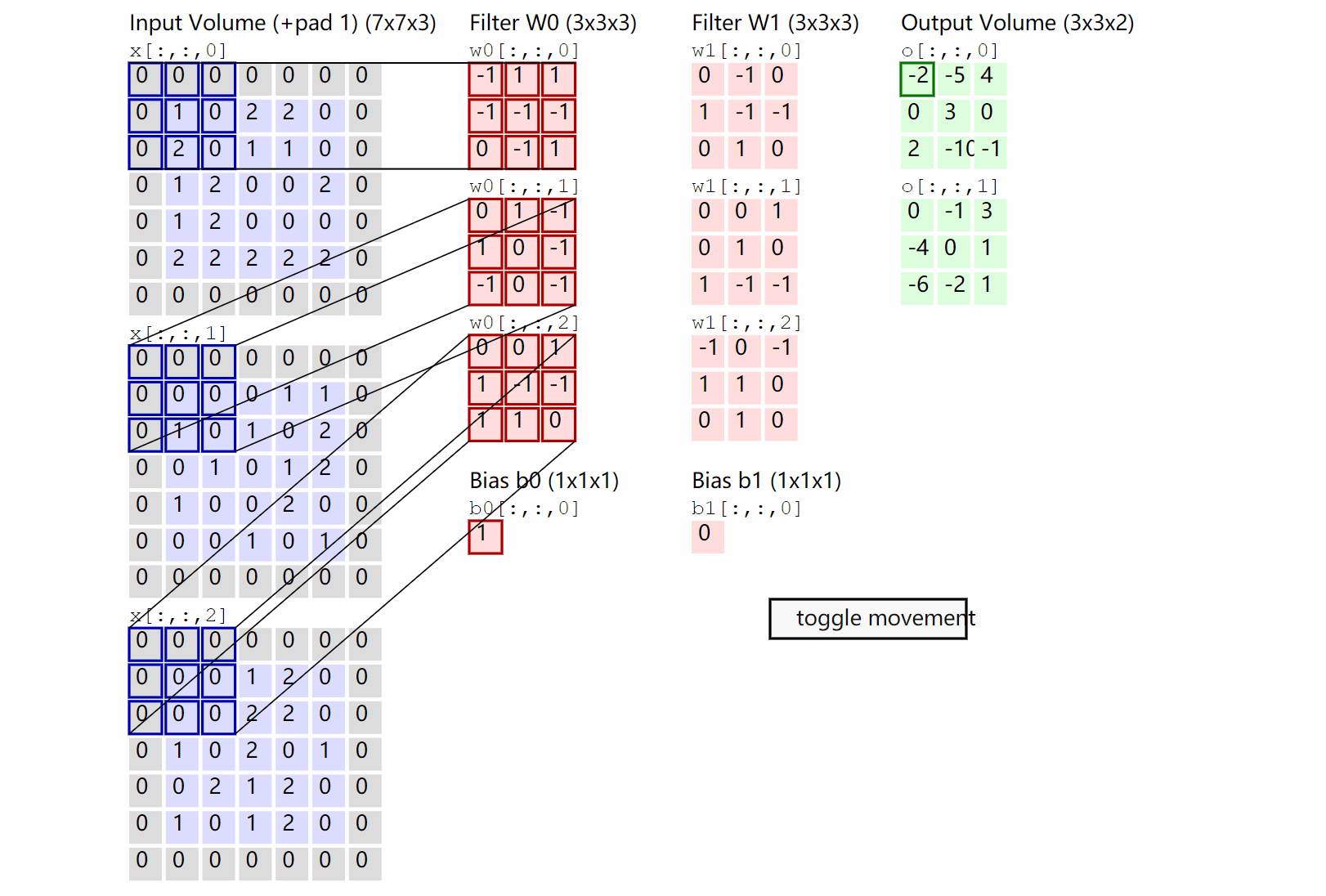$W_{2}=\left(W_{1}-F+2 P\right) / S+1\\ H_{2}=\left(H_{1}-F+2 P\right) / S+1 \\ D_{2}=K$

## Relu激励层

$\begin{equation}f(x)=\max (0, x)\end{equation}$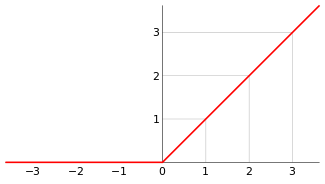Relu激励层的工作流程如下所示：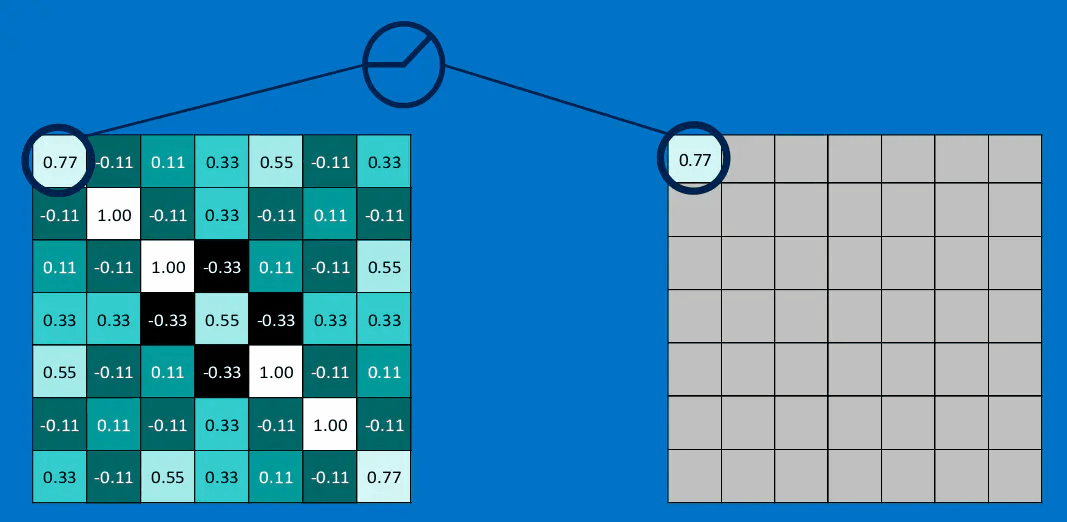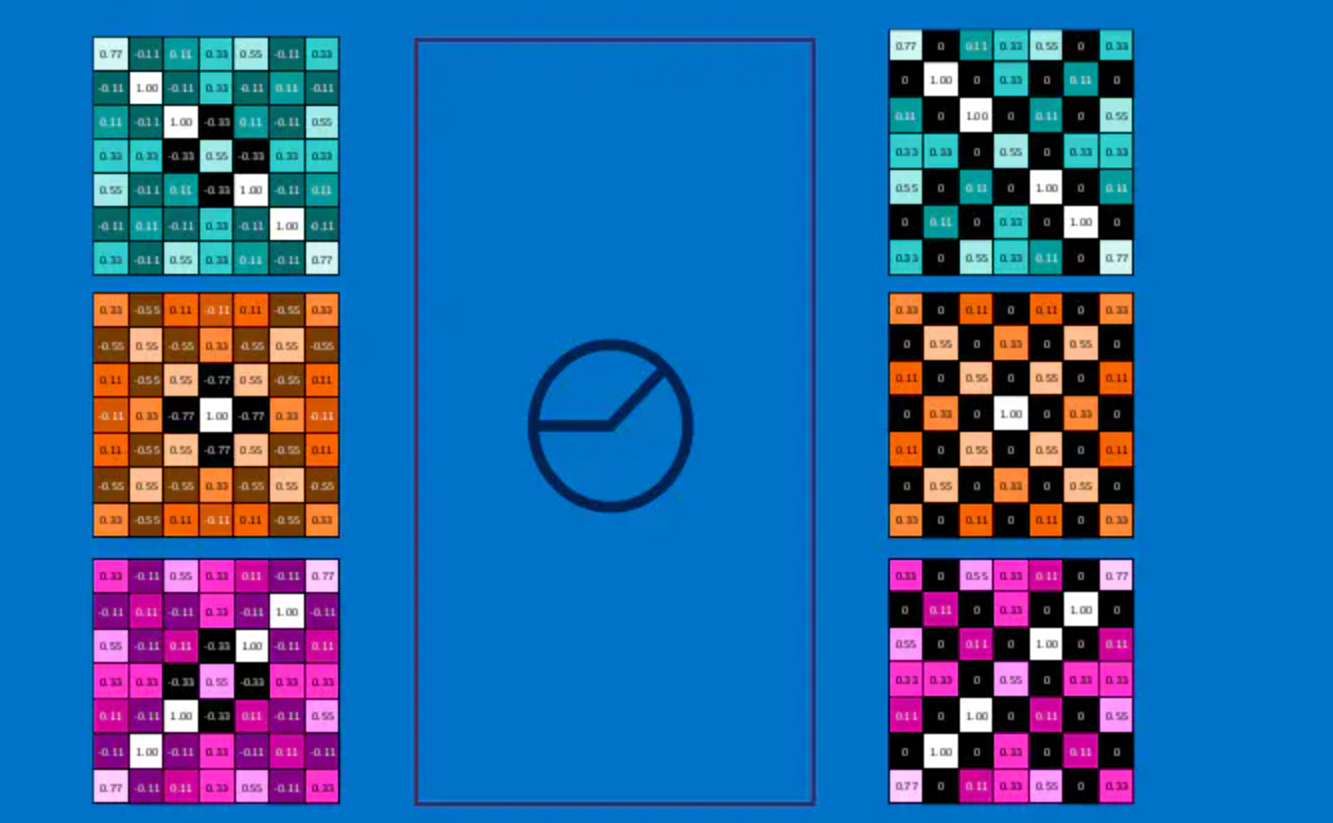## 池化（Pooling）

• 平均池化average pooling）：计算图像区域的平均值作为该区域池化后的值。
• 最大池化max pooling）：选图像区域的最大值作为该区域池化后的值。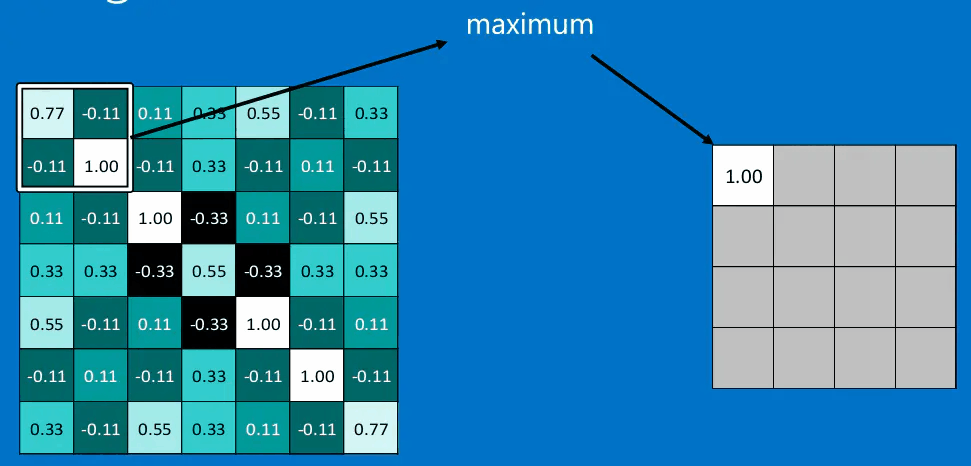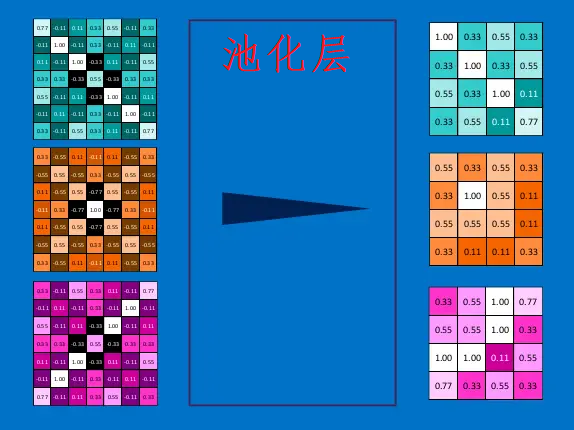\begin{equation}\begin{aligned} &W_{2}=\left(W_{1}-F\right) / S+1\\ &H_{2}=\left(H_{1}-F\right) / S+1\\ &D_{2}=D_{1} \end{aligned}\end{equation}

## 结合卷积层、激励层、池化层## 全连接层(Fully connected layers)